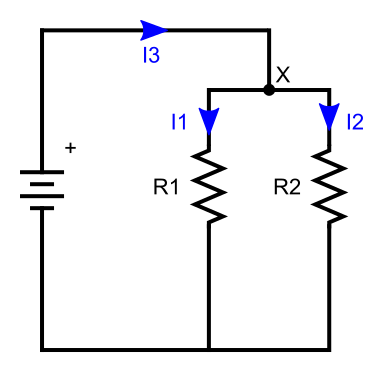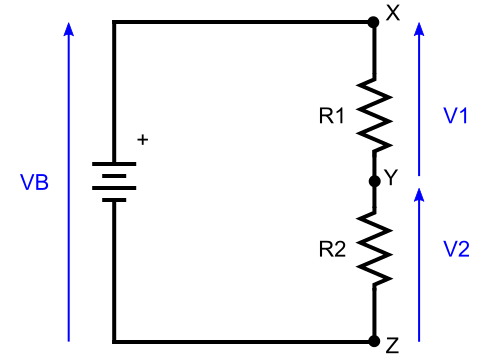# Kirchoffs's laws

Martin McBride, 2020-07-06
Tags battery resistor kirchoffs law
Categories electronics electricity

Kirchoff's two laws are possibly less well known that Ohm's law, but they are just as important. The three laws together allow us to solve networks of resistors.

## Kirchoff's first law

Kirchoff's first law, also known as Kirchoff's current law, states:

The algebraic sum of currents in a network of conductors meeting at a point is zero.

Here is a circuit that illustrates this:Expressed more simply, at any point in the circuit the total current flowing in must be equal to the total current flowing out. So for point X, this means that the incoming current I3 must be equal to the total outgoing current I1 + I2.

The convention is that incoming current (I3) is positive and outgoing current (I1 and I2) is negative, This means that the sum of (I1+I2+I3) will be zero.

## Kirchoff's second law

Kirchoff's second law, also known as Kirchoff's voltage law, states:

The directed sum of the voltages around any closed loop is zero.

Here is a circuit that illustrates this:In this case, the voltage law tells us that the battery voltage, VB, must equal the voltages across each resistor (V1 and V2). The directed sum mean we must take account of the direction of the voltage measurement. If we positive to mean that the voltage is measured in the clockwise direction, this means that VB is positive but V1 and V2 are negative, so the sum (VB+V1+V2) will be zero.

## Application of Kirchoff's laws - resistors in series

Let's take a look at this circuit in more detail:When two or more resistors (or any other components) are connected end to end, we say that they are connected in series.

It would be useful to know how much current flows round this circuit. We can use the three laws to find this out.

Firstly, we can apply Kirchoff's current law at point Y in the circuit. This law says that the current flowing into point Y is equal to tyhe current flowing out. In other words, the current that flows through R1 is equal to the current that flows through R2. This is intuitively fairly obvious, the current has nowhere else to go.

We will call this current I. Using Ohm's law we can relate this current to the resistance and voltage across each resistor:

I = V1/R1 = V2/R2


This equation gives us the relationship between V1 and V2:

V2 = R2*V1/R1


Using Kirchoff's voltage law, as we saw earlier:

VB = V1 + V2


So:

VB = V1 + R2*V1/R1 = V1*(1 + R2/R1) = V1*(R1 + R2)/R1


We can now find V1 in terms of the known quantities VB, R1 and R2:

V1 = VB*R1/(R1 + R2)


And solve for I:

I = V1*R1 = VB/(R1 + R2)


So the total current flowing through the circuit is equal to the voltage divided by the sum of the two resistors.

## Resistors in series

From the equation above, we can see that the effective resistance of R1 and R2 wired in series is given by R1+R2.

For example, if R1 was 100R and R2 was 50R, the total resistance of them both would be 150R.

## Voltage divider

The circuit above is sometimes used as a voltage divider. We can rearrange the equations above to show that the voltage at point Y is:

V2 = VB*R2/(R1 + R2)


So if we had a 6V battery, and again if R1 was 100R and R2 was 50R, the voltage at Y would be 2V.

## Application of Kirchoff's laws - resistors in parallel

Now let's take another look at the first circuit:When two or more resistors (or any other components) are connected side to side, we say that they are connected in parallel.

Again, it would be useful to know how much current flows round this circuit. This time Kirchoff's current law tells us that:

I3 = I1 + I2


Each resistor has the same voltage across it - the battery voltage VB. So we can find the current using Ohm's law:

I1 = VB/R1

I2 - VB/R2

I3 = VB* (1/R1 + 1/R2) = VB*(R1 + R2)/(R1*R2)


## Resistors in parallel

From the equations above we can see that the effective resistance of two resistors wired in parallel is:

R1*R2/(R1 + R2)


Copyright (c) Axlesoft Ltd 2021